# ISRO Scientist or Engineer Electronics 2012

Instructions

For the following questions answer them individually

Question 61

# For a Phase Locked Loop system, the open loop transfer function is G(s)=$$\frac{1000}{s (\frac{s}{1192}+1)}$$The phase margin is $$50^\circ$$ and the crossover frequency is approximately 1000 rad/s. A time delay is introduced in the phase detector reducing the phase margin to $$40^\circ$$ . The maximum permissible time delay ( in seconds)is :Question 62

# In this circuit, the relationship between $$V_{i} \& V_{o}$$, is: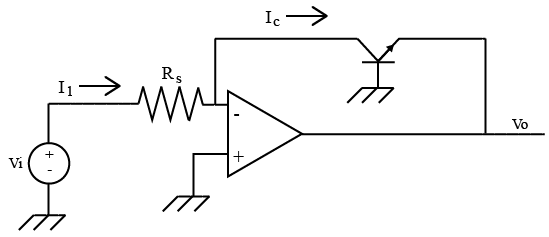Question 63

# Output Impedanceof a source follower is :Question 64

# For a negative feedback system shown in figure,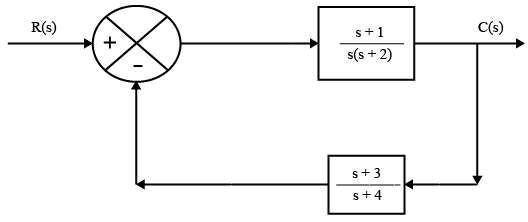the equivalent transfer function is :Question 65

# If $$(146)_{x}+(313)_{x-2}=(246)_{8}$$, then the value of base x isQuestion 66

# The truth table for implementing a boolean variable F is given by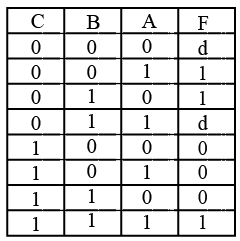where d represents don’t care states. The minimized expression for F isQuestion 67

# The state transition diagram for a sequence generator is shown in figure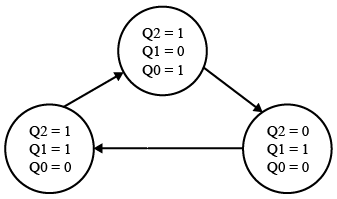It is designed using D F/Fs and combinational logic blocks $$L_{1}, L_{2}, \& L_{3}$$ and is initialized at $$(Q_{2} = 1 ,Q_{1} = 0, Q_{0} = 1)$$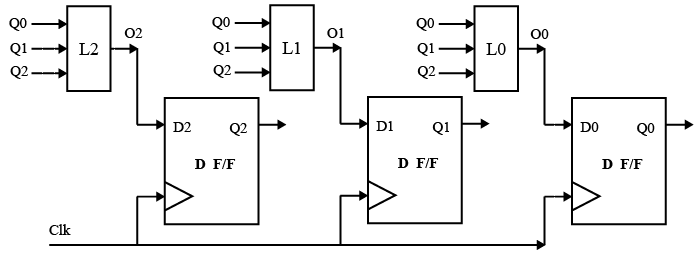The minimized expressions for O0, O1, & O2 are:Question 68

# The number of comparators needed in a 8-bit flash type A to D converterisQuestion 69

# The register in the 8085A that is used to keep track of the memory address of the next op-code to be run in the program is the :Question 70

# The Complement Accumulator (CMA) instruction of 8085 processor on execution affectsOR